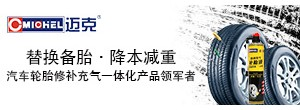# [变速器] 基于MATLAB的发动机曲轴扭振测试研究

### 亲，赶快注册吧，有更多精彩内容分享！

x
??1. 扭振角位移传感器的安装2.数据采样仪[B，A]=butter（N，Wn，‘low’）；

Y=filter（B，A，X）；abs(fft(X,N))；angle(fft(X,N))

Plot(k×D,abs(fftshift(X)),title(‘振幅频谱’))； %画幅特性

Plot(k×D,angle(fftshift(X)),title(‘相位频谱’))； %画相特性

n=4;

Fs=12 000;

LP=4 000;

[B,A]=butter(n,LP/Fs/2,'low')；

B = 0.2347?? 0.9388??? 1.4082??? 0.9388??? 0.2347

A =1.0000??? 1.3066??? 1.0305??? 0.3624??? 0.0558

 不错，支持一下

 本版积分规则 回帖并转播 回帖后跳转到最后一页